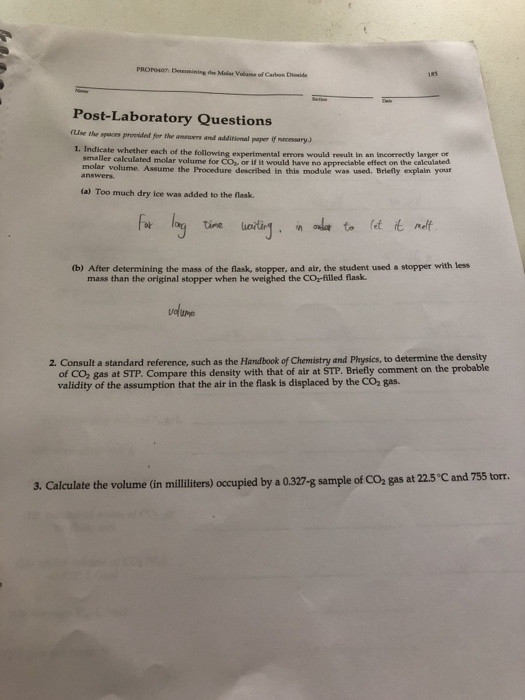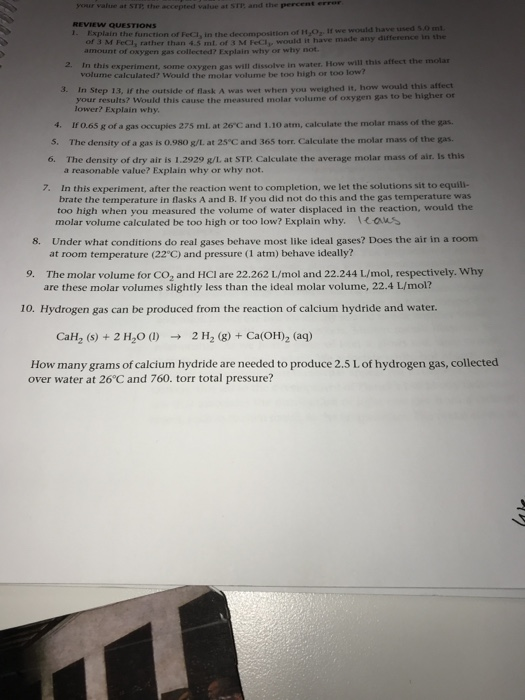# 1and 2 PROP407 Deenmining the Molar Volame of Carbon Dioside Post-Laboratory Questions ruse the spaces preedded...1and 2

PROP407 Deenmining the Molar Volame of Carbon Dioside Post-Laboratory Questions ruse the spaces preedded for the ansovers and additional paper if rancessaryJ 1. Indicate whether each of the tollowing experimental errors smaller calculated molar for molar volume. Assume the Procedure described in this module was used. Briefly explain your would result in an incorrectly larger ot Voume tor C0s, or if it would have no appreciable effect on the calculated o (a) Too much dry ice was added to the flask. tine uortign (b) After determining the mass of the flask, stopper, and air, the student used a stopper with less mass than the original stopper when he weighed the CO-filled flask 2. Consult a standard reference, such as the Handbook of Chemistry and Physics, to determine the density of CO2 gas at STP. Compare this density with that of air at STP. Briefly comment on the probable validity of the assumption that the air in the flask is displaced by the CO2 gas. 3. Calculate the volume (in milliliters) occupied by a 0.327-g sample of CO2 gas at 22.5'C and 755 tomr.

1)

a)

If Too much dry ice was added to the flask. It will make more CO2 gas and the volume will be higher than calculated. Then you will have an incorrect value.

b)

If the student use a stopper with less mass than the original stopper the increase in CO2 gas will not take place and the mass gained would not be correct, hence there was loss of mass from the less mass of stopper.

Therefore you will have an incorrect larger value for CO2 at the end of the experiment because mass of stopper will be less making the gain of the larger appear CO2 gas

#### Post as a guestwaa r the accepted value at SIF, and the perce REVIEW QUESTIONS MPC, rather than 45 ml of Me would have made any difference in the amount of oxygen was collected? Explain why or why not In this experiment, some oxy en gas will dissolve in water How will this affect the molar volume calculated? Would the molar volume be too high or too low In Step 1, if the outside of flask was wet when you weighed it, how...